### Example 41.5 Joint Modeling of Binary and Count Data

Clustered data arise when multiple observations are collected on the same sampling or experimental unit. Often, these multiple observations refer to the same attribute measured at different points in time or space. This leads to repeated measures, longitudinal, and spatial data, which are special forms of multivariate data. A different class of multivariate data arises when the multiple observations refer to different attributes.

The data set hernio, created in the following DATA step, provides an example of a bivariate outcome variable. It reflects the condition and length of hospital stay for 32 herniorrhaphy patients. These data are based on data given by Mosteller and Tukey (1977) and reproduced in Hand et al. (1994, pp. 390, 391). The data set that follows does not contain all the covariates given in these sources. The response variables are leave and los; these denote the condition of the patient upon leaving the operating room and the length of hospital stay after the operation (in days). The variable leave takes on the value one if a patient experiences a routine recovery, and the value zero if postoperative intensive care was required. The binary variable OKstatus distinguishes patients based on their postoperative physical status (1 implies better status).

data hernio;
input patient age gender$OKstatus leave los; datalines; 1 78 m 1 0 9 2 60 m 1 0 4 3 68 m 1 1 7 4 62 m 0 1 35 5 76 m 0 0 9 6 76 m 1 1 7 7 64 m 1 1 5 8 74 f 1 1 16 9 68 m 0 1 7 10 79 f 1 0 11 11 80 f 0 1 4 12 48 m 1 1 9 13 35 f 1 1 2 14 58 m 1 1 4 15 40 m 1 1 3 16 19 m 1 1 4 17 79 m 0 0 3 18 51 m 1 1 5 19 57 m 1 1 8 20 51 m 0 1 8 21 48 m 1 1 3 22 48 m 1 1 5 23 66 m 1 1 8 24 71 m 1 0 2 25 75 f 0 0 7 26 2 f 1 1 0 27 65 f 1 0 16 28 42 f 1 0 3 29 54 m 1 0 2 30 43 m 1 1 3 31 4 m 1 1 3 32 52 m 1 1 8 ;  While the response variable los is a Poisson count variable, the response variable leave is a binary variable. You can perform separate analysis for the two outcomes, for example, by fitting a logistic model for the operating room exit condition and a Poisson regression model for the length of hospital stay. This, however, would ignore the correlation between the two outcomes. Intuitively, you would expect that the length of postoperative hospital stay is longer for those patients who had more tenuous exit conditions. The following DATA step converts the data set hernio from the multivariate form to the univariate form. In the multivariate form the responses are stored in separate variables. The GLIMMIX procedure requires the univariate data structure. data hernio_uv; length dist$7;
set hernio;
response = (leave=1);
dist     = "Binary";
output;
response = los;
dist     = "Poisson";
output;
keep patient age OKstatus response dist;
run;


This DATA step expands the 32 observations in the data set hernio into 64 observations, stacking two observations per patient. The character variable dist identifies the distribution that is assumed for the respective observations within a patient. The first observation for each patient corresponds to the binary response.

The following GLIMMIX statements fit a logistic regression model with two regressors (age and OKStatus) to the binary observations:

proc glimmix data=hernio_uv(where=(dist="Binary"));
model response(event='1') = age OKStatus / s dist=binary;
run;


The EVENT=(’1’) response option requests that PROC GLIMMIX model the probability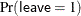—that is, the probability of routine recovery. The fit statistics and parameter estimates for this univariate analysis are shown in Output 41.5.1. The coefficient for the age effect is negative (–0.07725) and marginally significant at the 5% level (p = 0.0491). The negative sign indicates that the probability of routine recovery decreases with age. The coefficient for the OKStatus variable is also negative. Its large standard error and the p-value of 0.7341 indicate, however, that this regressor is not significant.

Output 41.5.1: Univariate Logistic Regression

The GLIMMIX Procedure

Fit Statistics
-2 Log Likelihood 32.77
AIC (smaller is better) 38.77
AICC (smaller is better) 39.63
BIC (smaller is better) 43.17
CAIC (smaller is better) 46.17
HQIC (smaller is better) 40.23
Pearson Chi-Square 30.37
Pearson Chi-Square / DF 1.05

Parameter Estimates
Effect Estimate Standard Error DF t Value Pr > |t|
Intercept 5.7694 2.8245 29 2.04 0.0503
age -0.07725 0.03761 29 -2.05 0.0491
OKstatus -0.3516 1.0253 29 -0.34 0.7341

Based on the univariate logistic regression analysis, you would probably want to revisit the model, examine other regressor variables, test for gender effects and interactions, and so forth. The two-regressor model is sufficient for this example. It is illustrative to trace the relative importance of the two regressors through various types of models.

The next statements fit the same regressors to the count data:

proc glimmix data=hernio_uv(where=(dist="Poisson"));
model response = age OKStatus / s dist=Poisson;
run;


For this response, both regressors appear to make significant contributions at the 5% significance level (Output 41.5.2). The sign of the coefficient seems appropriate; the length of hospital stay should increase with patient age and be shorter for patients with better preoperative health. The magnitude of the scaled Pearson statistic (4.48) indicates, however, that there is considerable overdispersion in this model. This could be due to omitted variables or an improper distributional assumption. The importance of preoperative health status, for example, can change with a patient’s age, which could call for an interaction term.

Output 41.5.2: Univariate Poisson Regression

The GLIMMIX Procedure

Fit Statistics
-2 Log Likelihood 215.52
AIC (smaller is better) 221.52
AICC (smaller is better) 222.38
BIC (smaller is better) 225.92
CAIC (smaller is better) 228.92
HQIC (smaller is better) 222.98
Pearson Chi-Square 129.98
Pearson Chi-Square / DF 4.48

Parameter Estimates
Effect Estimate Standard Error DF t Value Pr > |t|
Intercept 1.2640 0.3393 29 3.72 0.0008
age 0.01525 0.004454 29 3.42 0.0019
OKstatus -0.3301 0.1562 29 -2.11 0.0433

You can also model both responses jointly. The following statements request a multivariate analysis:

proc glimmix data=hernio_uv;
class dist;
model response(event='1') = dist dist*age dist*OKstatus /
noint s dist=byobs(dist);
run;


The DIST=BYOBS option in the MODEL statement instructs the GLIMMIX procedure to examine the variable dist in order to identify the distribution of an observation. The variable can be character or numeric. See the DIST= option of the MODEL statement for a list of the numeric codes for the various distributions that are compatible with the DIST=BYOBS formulation. Because no LINK= option is specified, the link functions are chosen as the default links that correspond to the respective distributions. In this case, the logit link is applied to the binary observations and the log link is applied to the Poisson outcomes. The dist variable is also listed in the CLASS statement, which enables you to use interaction terms in the MODEL statement to vary the regression coefficients by response distribution. The NOINT option is used here so that the parameter estimates of the joint model are directly comparable to those in Output 41.5.1 and Output 41.5.2.

The Fit Statistics and Parameter Estimates tables of this bivariate estimation process are shown in Output 41.5.3.

Output 41.5.3: Bivariate Analysis -- Independence

The GLIMMIX Procedure

Fit Statistics
Description Binary Poisson Total
-2 Log Likelihood 32.77 215.52 248.29
AIC (smaller is better) 44.77 227.52 260.29
AICC (smaller is better) 48.13 230.88 261.77
BIC (smaller is better) 53.56 236.32 273.25
CAIC (smaller is better) 59.56 242.32 279.25
HQIC (smaller is better) 47.68 230.44 265.40
Pearson Chi-Square 30.37 129.98 160.35
Pearson Chi-Square / DF 1.05 4.48 2.76

Parameter Estimates
Effect dist Estimate Standard Error DF t Value Pr > |t|
dist Binary 5.7694 2.8245 58 2.04 0.0456
dist Poisson 1.2640 0.3393 58 3.72 0.0004
age*dist Binary -0.07725 0.03761 58 -2.05 0.0445
age*dist Poisson 0.01525 0.004454 58 3.42 0.0011
OKstatus*dist Binary -0.3516 1.0253 58 -0.34 0.7329
OKstatus*dist Poisson -0.3301 0.1562 58 -2.11 0.0389

The Fit Statistics table now contains a separate column for each response distribution, as well as an overall contribution. Because the model does not specify any random effects or R-side correlations, the log likelihoods are additive. The parameter estimates and their standard errors in this joint model are identical to those in Output 41.5.1 and Output 41.5.2. The p-values reflect the larger sample size in the joint analysis. Note that the coefficients would be different from the separate analyses if the dist variable had not been used to form interactions with the model effects.

There are two ways in which the correlations between the two responses for the same patient can be incorporated. You can induce them through shared random effects or model the dependency directly. The following statements fit a model that induces correlation:

proc glimmix data=hernio_uv;
class patient dist;
model response(event='1') = dist dist*age dist*OKstatus /
noint s dist=byobs(dist);
random int / subject=patient;
run;


Notice that the patient variable has been added to the CLASS statement and as the SUBJECT= effect in the RANDOM statement.

The Fit Statistics table in Output 41.5.4 no longer has separate columns for each response distribution, because the data are not independent. The log (pseudo-)likelihood does not factor into additive component that correspond to distributions. Instead, it factors into components associated with subjects.

Output 41.5.4: Bivariate Analysis -- Mixed Model

The GLIMMIX Procedure

Fit Statistics
-2 Res Log Pseudo-Likelihood 226.71
Generalized Chi-Square 52.25
Gener. Chi-Square / DF 0.90

Covariance Parameter Estimates
Cov Parm Subject Estimate Standard Error
Intercept patient 0.2990 0.1116

Solutions for Fixed Effects
Effect dist Estimate Standard Error DF t Value Pr > |t|
dist Binary 5.7783 2.9048 29 1.99 0.0562
dist Poisson 0.8410 0.5696 29 1.48 0.1506
age*dist Binary -0.07572 0.03791 29 -2.00 0.0552
age*dist Poisson 0.01875 0.007383 29 2.54 0.0167
OKstatus*dist Binary -0.4697 1.1251 29 -0.42 0.6794
OKstatus*dist Poisson -0.1856 0.3020 29 -0.61 0.5435

The estimate of the variance of the random patient intercept is 0.2990, and the estimated standard error of this variance component estimate is 0.1116. There appears to be significant patient-to-patient variation in the intercepts. The estimates of the fixed effects as well as their estimated standard errors have changed from the bivariate-independence analysis (see Output 41.5.3). When the length of hospital stay and the postoperative condition are modeled jointly, the preoperative health status (variable OKStatus) no longer appears significant. Compare this result to Output 41.5.3; in the separate analyses the initial health status was a significant predictor of the length of hospital stay. A further joint analysis of these data would probably remove this predictor from the model entirely.

A joint model of the second kind, where correlations are modeled directly, is fit with the following GLIMMIX statements:

proc glimmix data=hernio_uv;
class patient dist;
model response(event='1') = dist dist*age dist*OKstatus /
noint s dist=byobs(dist);
random _residual_ / subject=patient type=chol;
run;


Instead of a shared G-side random effect, an R-side covariance structure is used to model the correlations. It is important to note that this is a marginal model that models covariation on the scale of the data. The previous model involves the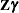random components inside the linear predictor.

The _RESIDUAL_ keyword instructs PROC GLIMMIX to model the R-side correlations. Because of the SUBJECT=PATIENT option, data from different patients are independent, and data from a single patient follow the covariance model specified with the TYPE= option. In this case, a generally unstructuredcovariance matrix is modeled, but in its Cholesky parameterization. This ensures that the resulting covariance matrix is at least positive semidefinite and stabilizes the numerical optimizations.

Output 41.5.5: Bivariate Analysis – Marginal Correlated Error Model

The GLIMMIX Procedure

Fit Statistics
-2 Res Log Pseudo-Likelihood 240.98
Generalized Chi-Square 58.00
Gener. Chi-Square / DF 1.00

Covariance Parameter Estimates
Cov Parm Subject Estimate Standard Error
CHOL(1,1) patient 1.0162 0.1334
CHOL(2,1) patient 0.3942 0.3893
CHOL(2,2) patient 2.0819 0.2734

Solutions for Fixed Effects
Effect dist Estimate Standard Error DF t Value Pr > |t|
dist Binary 5.6514 2.8283 26 2.00 0.0563
dist Poisson 1.2463 0.7189 26 1.73 0.0948
age*dist Binary -0.07568 0.03765 26 -2.01 0.0549
age*dist Poisson 0.01548 0.009432 26 1.64 0.1128
OKstatus*dist Binary -0.3421 1.0384 26 -0.33 0.7445
OKstatus*dist Poisson -0.3253 0.3310 26 -0.98 0.3349

The Covariance Parameter Estimates table in Output 41.5.5 contains three entries for this model, corresponding to a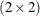covariance matrix for each patient. The Cholesky root of thematrix is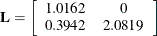so that the covariance matrix can be obtained as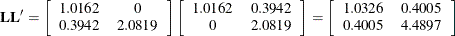This is not the covariance matrix of the data, however, because the variance functions need to be accounted for.

The p-values in the Solutions for Fixed Effects table indicate the same pattern of significance and nonsignificance as in the conditional model with random patient intercepts.